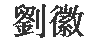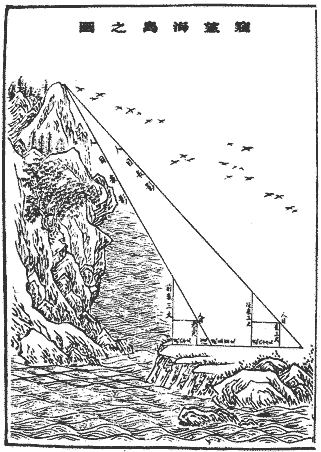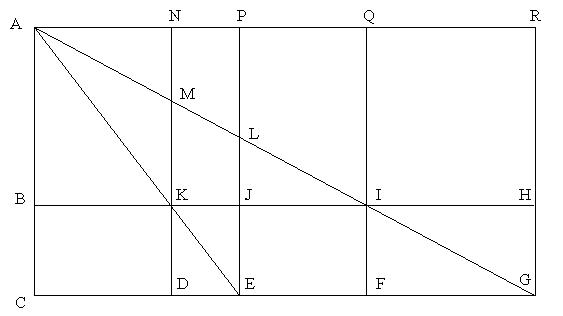Math 4005: GeometryThe Sea Island Mathematical Manual

Introduction. The Sea Island Mathematical Manual (Haidao Suanjing,) was composed in A.D.263 by the Chinese scholar-official Liu Hui (). Originally, it was part of a commentary on an earleir mathematical work, the Jiuzhang Suanshu, or The Nine Chapters on the Mathematical Art, an official handbook used by the ruling civil bureaucracy. Liu felt that the treatment of certain surveying problems was inadequate. Therefore, he composed nine problems of his own and described how to solve them. Each problem involved measurement of distant or inaccessible objects by triangulation.

Liu's work was made into a separate manual early in the Tang dynasty (A.D. 618-906), and it became part of the curriculum of study in the Chinese Royal Academy in A.D. 656. The title of the manual comes from the first problem, which is to measure the height of a peak on an off-shore island. A translation extensive information about Liu's work can be found in: Swetz, F. (1992)."The Sea Island Mathematical Manual": Surveying and Mathematics in Ancient China. University Park: The Pennsylvania State University Press 1992.

The problem.

The first problem in the Sea Island Mathematical Manual is as follows. (The translation is quoted from from Swetz, op. cit.)

Now for [the purpose of] looking at a sea island, erect two poles of the same height, 3 zhang [on the ground], the distance between the front and rear [pole] being a thousand bu. Assume that the rear pole is aligned with the front pole. Move away 123 bu from the front pole and observe the peak of the island from ground level; it is seen that the tip of the front pole coincides with the peak. Move backward 127 bu from the rear pole and observe the peak of the island from ground level again; the tip of the back pole also coincides with the peak.

What is the height of the island and how far is it from the pole?Comments. The diagram below shows the basic set-up of the Sea Island problem. Lines that appear parallel are parallel. Point A is the top of a mountain on an island out at sea. Point C is at sea-level, directly below A. A surveyor on the mainland (DG) wishes to determine the height of the mountain |CA|. However, she cannot get to any of the points A, B or C. At best, she can find the distances between points in the set, {D, E, F, G, H, I, J, K}.Problem. In the diagram above, suppose |KD| = 5, |DE| = 4, |DF| = 12 and |DG| = 28. What is |AC|?

The following questions are intended as scaffolding to take you deeper into this problem.

1. What angles in the above figure are congruent? What triangles are similar? What proportions are implicit in the diagram?
2. Suppose lengths |KD|, |DE|, |DF| and |DG| are known. How can you use this information to determine |AC|?
3. There are numerous procedures that one might follow to compute |AC| from measurements you might make between points whose names appear in the INSIDE the large rectangle. Challenge: describe as many different methods as you can.
4. Is it not necessary to know all the measurements listed in the previous question in order to calculate |AC|. Suppose you can measure between any two points whose names appear in the INSIDE the large rectangle. What would be the least number of measurements needed in order to provide enough data to find |AC|? What would be the easiest procedure?
5. Suppose you know the ratio of |KI| to |EG| and the length |IF|. Can you find |AC|? Explain.